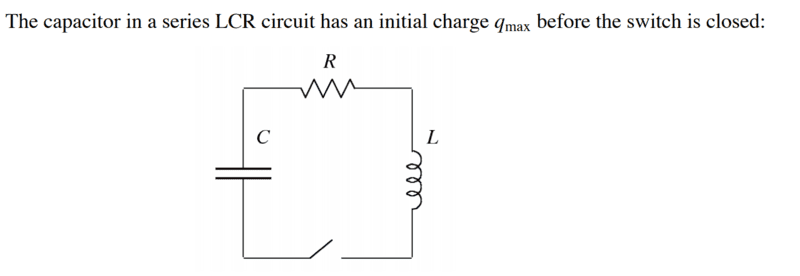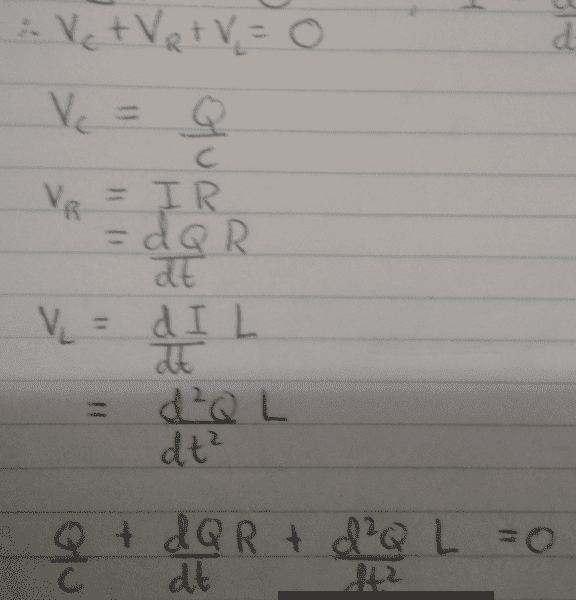Resonance and RCL circuits

• Engineering
physicsStudent00

Homework Statement

I'm just really lost on this topic and honestly don't have too much of an idea.(a) Using Kirchoff's loop rule, find the differential equation satisfied by the charge q(t).

(b) Verify that q(t) = q_max e^-(a/t) cos(ωt) satisfies the differential equation for particular values of a and w and find these values.

(c) The resistance R_c for which w = 0 yields critical damping. If L = 10 mH and C = 0.2 mF, determine R_c.

(d) Sketch q(tL)for R < R_c, R = R_c, and R > R_c.

The Attempt at a Solution

a) using the loop law all voltages must add to give 0 so you use an equation that includes q for each of the voltages, giving
q/t+dq/dt*R+d^2q/dt^2*L=0

b) so if we are to solve you place it at time =0 because we want q(t)=0? therefore q(t) = q_max*e^-(a/t) and solve for "a" that way. but what original value of q(t) would be used, on top of this how would i then solve for ω.

c) i think the equation ω_0=1/√LC is used but i don't know how that translates the the question as it uses ω and not ω_0.

d) it should be exponential but i've been told me someone who is always right that i'm wrong.

any help would be greatly appreciated as im really lost.

Orodruin
Staff Emeritus
Homework Helper
Gold Member
2021 Award
using the loop law all voltages must add to give 0 so you use an equation that includes q for each of the voltages, giving
q/t+dq/dt*R+d^2q/dt^2*L=0
No, this is incorrect. For example, what happened to the capacitance C? Also, you need to be more careful about what signs your terms have and make sure that they all have the same physical dimension.

•physicsStudent00
physicsStudent00
No, this is incorrect. For example, what happened to the capacitance C? Also, you need to be more careful about what signs your terms have and make sure that they all have the same physical dimension.
thanks for the help,
so how should i best approach this question then

Orodruin
Staff Emeritus
Homework Helper
Gold Member
2021 Award
so how should i best approach this question then
In the way you prescribed, by going around the loop and making sure that the voltages add up to zero. However, you need to be more careful while doing so so that you get the correct result. Can you write down your argumentation for each of the terms?

physicsStudent00
In the way you prescribed, by going around the loop and making sure that the voltages add up to zero. However, you need to be more careful while doing so so that you get the correct result. Can you write down your argumentation for each of the terms?
this is my exact working, im not exactly the best at using the computer for showing my workingOrodruin
Staff Emeritus
Homework Helper
Gold Member
2021 Award
This still does not explain your reasoning for the signs of each term. In which direction have you defined the current I? How does this relate to the potential drop across the resistor/inductor? What happens to the charge on the capacitor when you have a positive current I? (This will depend on the direction you defined as positive for I.)

physicsStudent00
This still does not explain your reasoning for the signs of each term. In which direction have you defined the current I? How does this relate to the potential drop across the resistor/inductor? What happens to the charge on the capacitor when you have a positive current I? (This will depend on the direction you defined as positive for I.)
i honestly have no idea, i'm really struggling with this whole topic in general.

donpacino
Gold Member
There is a sign mistake. Draw your circuit on a piece of paper. Draw the direction I is going. Then knowing the direction re-write your equations. Either 1, or 2 of the signs will change if you do it right.

•physicsStudent00
donpacino
Gold Member
Think about what a voltage DROP across a resistor means

•physicsStudent00
physicsStudent00
ok i know see what you mean, as by saying that all the voltages are adding it means each is creating a voltage which is clearly wrong. across the resistor there should be a drop in voltage so the sign will be negative, however across L and C it would have a positive sign as they both drive a charge, hence current and voltage?

donpacino
Gold Member
ok i know see what you mean, as by saying that all the voltages are adding it means each is creating a voltage which is clearly wrong. across the resistor there should be a drop in voltage so the sign will be negative, however across L and C it would have a positive sign as they both drive a charge, hence current and voltage?
not quite.... technically the voltage is dropped across the inductor too, my hint was only a partial hint, so slightly misleading

•physicsStudent00
physicsStudent00
not quite.... technically the voltage is dropped across the inductor too, my hint was only a partial hint, so slightly misleading
ok so starting at the capacitor and moving around the loop clockwise the sum of the voltages could be written as
V_c - V_r -V_l =0

Last edited by a moderator:
donpacino
Gold Member
ok so starting at the capacitor and moving around the loop clockwise the sum of the voltages could be written as
V_c - V_r -V_l =0
Yup, do you understand why?

physicsStudent00
Yup, do you understand why?
yeah i get it now, i just wasn't looking at the loop voltage law too literally. so the voltage the would be increased across the capacitor, then be diminished across the inductor and resistor so that it goes back to zero